# GMAT Math : DSQ: Calculating the surface area of a tetrahedron

## Example Questions

### Example Question #1 : Dsq: Calculating The Surface Area Of A Tetrahedron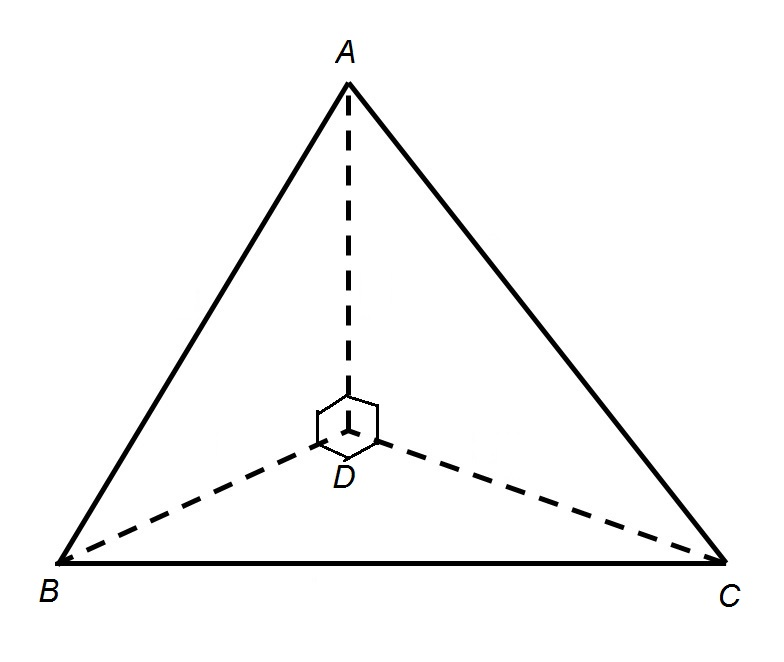Note: Figure NOT drawn to scale.

Refer to the above tetrahedron or triangular pyramid.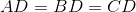.

Calculate the surface area of the tetrahedron.

Statement 1: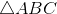has perimeter 60.

Statement 2: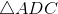has area 100.

Statement 1 ALONE is sufficient to answer the question, but Statement 2 ALONE is NOT sufficient to answer the question.

BOTH statements TOGETHER are insufficient to answer the question.

Statement 2 ALONE is sufficient to answer the question, but Statement 1 ALONE is NOT sufficient to answer the question.

EITHER statement ALONE is sufficient to answer the question.

BOTH statements TOGETHER are sufficient to answer the question, but NEITHER statement ALONE is sufficient to answer the question.

EITHER statement ALONE is sufficient to answer the question.

Explanation: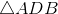, and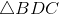, all being right triangles with the same leg lengths, are congruent, and, consequently, their diagonals are congruent, makingequilateral.

Assume Statement 1 alone. The sidelength of equilateralis one third of its perimeter of 60, or 20. This is also the common length of the hypotenuses of isoseles right triangles, and, so by the 45-45-90 Theorem, each leg length can be computed by dividing 20 by: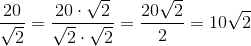Assume Statement 2 alone.has area 100; since the area of a right triangle is half the product of the lengths of its legs, we can find the common leg length using this formula: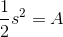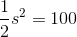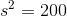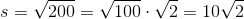This is the common length of the legs of the three right triangles; by the 45-45-90 Theorem, each hypotenuse - and each side of equilateral- istimes this, or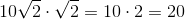.

Therefore, from either statement alone, the lengths of all sides of the tetrahedron can be found, and the area formulas for a right triangle and an equilateral can be applied to find the areas of all four faces.

### Example Question #2 : Dsq: Calculating The Surface Area Of A Tetrahedron

What is the surface area of the tetrahedron?

1. The length of an edge measures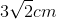.
2. The volume of the tetrahedron is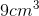.

Statement 2 alone is sufficient, but statement 1 alone is not sufficient to answer the question.

Statements 1 and 2 are not sufficient, and additional data is needed to answer the question.

Statement 1 alone is sufficient, but statement 2 alone is not sufficient to answer the question.

Both statements taken together are sufficient to answer the question, but neither statement alone is sufficient.

Each statement alone is sufficient to answer the question.

Each statement alone is sufficient to answer the question.

Explanation:

The surface area of a tetrahedron is found by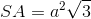whererepresents the edge value.

Situation 1: We're given ourvalue so we just need to plug it into our equation.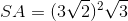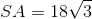Situation 2: We use the given volume to solve for the length of the edge.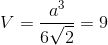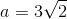Now that we have a length, we can plug it into the equation for the surface area: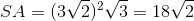Thus, each statement alone is sufficient to answer the question.

Tired of practice problems?

Try live online GMAT prep today.